Home > CALC > Chapter 10 > Lesson 10.1.7 > Problem10-70

10-70.
1. Examine the following series. Use tests that you have learned so far to determine if the series converges or diverges. Name the tests that you use. Homework Help ✎

1.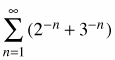2.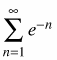3.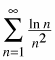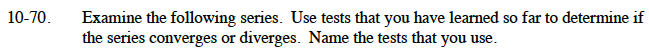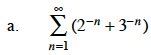This is the sum of two geometric series. Do both of these series converge?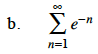This is a geometric series. What is the value of r?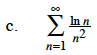$\sqrt{n}>\ln(n)$

$\frac{\sqrt{n}}{n^2}=\frac{1}{n^{1.5}}>\frac{\ln(n)}{n^2}$